Share

# Selina solutions for Concise Physics Class 10 ICSE chapter 1 - Force [Latest edition]

Course
Textbook page

#### Chapters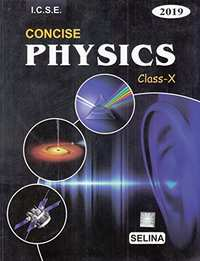## Chapter 1: Force

Exercise -1 (A)Exercise - 1 (A)OthersExercise -1(A)Exercise - 1(A)Exercise - 1 (B)Exercise - 1 (C)

#### Selina solutions for Concise Physics Class 10 ICSE Chapter 1 Force Exercise Exercise -1 (A), Exercise - 1 (A), Exercise -1(A), Exercise - 1(A) [Pages 9 - 10]

Exercise -1 (A) | Q 1.1 | Page 9

State the condition when on applying a force, the body has:

the translational motion

Exercise - 1 (A) | Q 1.2 | Page 9

State the condition when on applying a force, the body has:

the rotational motion.

Q 2 | Page 9

Define moment of force and state its S.I unit.

Exercise -1 (A) | Q 3 | Page 9

State whether the moment of force is a scalar or vector quantity?

Exercise - 1 (A) | Q 4 | Page 9

State two factors affecting the turning effect of a force.

Exercise -1 (A) | Q 5 | Page 10

When does a body rotate? State one way to change the direction of rotation of a body. Given a suitable example to explain your answer.

Exercise -1 (A) | Q 6 | Page 10

Write the expression for the moment of force about a given axis.

Exercise -1(A) | Q 7 | Page 10

What do you understand by the clockwise and anticlockwise moment of force? When is it taken positive?

Exercise - 1 (A) | Q 8 | Page 10

State one way to reduce the movement of given force about a given axis of rotation.

Exercise -1 (A) | Q 9 | Page 10

State one way to obtain greater moment of a given force about a given axis of rotation.

Exercise - 1 (A) | Q 10 | Page 10

Why is it easier to open a door by applying the force at the free end of it?

Exercise - 1(A) | Q 11 | Page 10

The stone of hand flour grinder is provided with a handle near its rim. Give reason.

Exercise - 1 (A) | Q 12 | Page 10

It is easier to turn the steering wheel of a large diameter than that of a small diameter. Given reason.

Exercise - 1 (A) | Q 13 | Page 10

A spanner (or wrench) has a long handle. Why?

Exercise - 1 (A) | Q 14 | Page 10

A jack screw is provided with a long arm. Explain why?

Exercise - 1 (A) | Q 15 | Page 10

A, B and C are the three forces each of magnitude 4 n acting in the plane of the paper as shown in Figure. The point O lies in the same plane.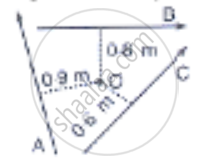1) Which force has the least moment about O? Give a reason.

2) which force has the greatest moment about O? Give a reason.

3) Name the forces producing (a) Clockwise (b) anticlockwise moments.

4) what is the resultant torque about the point O?

Exercise - 1 (A) | Q 16 | Page 10

The adjacent diagram shows a heavy roller, with its axle at O. which its axle at O. which is to be raised on a pavement XY by applying a minimum possible force. show by an arrow on the diagram the point of application and the direction in which the force should be applied.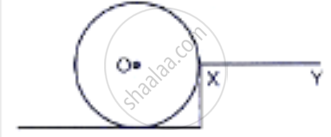Exercise - 1 (A) | Q 17.1 | Page 10

A body is acted upon by two force of magnitude F but in opposite direction. State the effect of the force if

both forces act at the same point of the body.

Exercise - 1 (A) | Q 17.2 | Page 10

A body is acted upon by two force of magnitude F, but in opposite directions. State the effect of the force if

the two forces act at two different points of the body at a separation r.

Exercise - 1 (A) | Q 18 | Page 10

Draw a neat labelled diagram to show the direction of two forces acting on a body to produce rotation in it. Also, mark the point about which rotation takes place by the letter O.

Exercise - 1 (A) | Q 19.1 | Page 10

What do you understand by the term couple?

Exercise - 1 (A) | Q 19.2 | Page 10

State the couple effect. Give two example of couple action in our daily life.

Exercise - 1 (A) | Q 20 | Page 10

Define moment of couple. Write its S.I unit

Exercise - 1 (A) | Q 21 | Page 10

Prove that Moment of couple = Force x couple arm.

Exercise - 1 (A) | Q 22 | Page 10

What do you mean by an equilibrium of a body?

Exercise - 1 (A) | Q 23.1 | Page 10

State the condition when a body is in static equilibrium. Give one example of static equilibrium.

Exercise - 1 (A) | Q 23.2 | Page 10

State the condition when a body is in dynamic equilibrium. Give one example of dynamic equilibrium.

Exercise - 1 (A) | Q 24 | Page 10

State two condition for a body acted upon by several forces to be in equilibrium.

Exercise - 1 (A) | Q 25 | Page 10

State the principle of moments. Give one device as an application of it

Exercise - 1 (A) | Q 26 | Page 10

Describe a simple experiment to verify the principle of moments, if you are supplied with a metre rule, a fulcrum and two springs with slotted weights.

Exercise - 1 (A) | Q 27.1 | Page 10

Complete the following sentence :

The S.I. unit of moment of force is _________.

Exercise - 1 (A) | Q 27.2 | Page 10

Complete the following sentence :

In equilibrium algebraic sum of moments of all forces about the point of rotation is ______________.

Exercise - 1 (A) | Q 27.3 | Page 10

Complete the following sentence :

In a beam balance when the beam is balanced in a horizontal position, it is in ____________equilibrium.

Exercise - 1 (A) | Q 27.4 | Page 10

Complete the following sentence :

The moon revolving around the earth is in ____________ equilibrium.

#### Selina solutions for Concise Physics Class 10 ICSE Chapter 1 Force Exercise Exercise - 1 (A) [Pages 10 - 11]

Exercise - 1 (A) | Q 1 | Page 10

Multiple choice Type:

The moment of a force about axis depends:

• Only on the magnitude of force

• Only on the perpendicular distance of force from the axis

• Neither on the force nor on the perpendicular distance of force from the axis

• Both on the force and its perpendicular distance from the axis.

Exercise - 1 (A) | Q 2 | Page 11

Multiple choice Type:

A body is acted upon by two unequal forces in opposite directions, but not in the same line. The effect is that:

• The body will have only the rotational motion

• The body will have only the translational motion

• The body will have neither the rotational motion nor the translational motion

• The body will have rotational as well as translational motion.

#### Selina solutions for Concise Physics Class 10 ICSE Chapter 1 Force Exercise Exercise - 1 (A) [Pages 11 - 12]

Exercise - 1 (A) | Q 1 | Page 11

The moment of a force of 10 N about a fixed point O is 5 N m. Calculate the distance of the point O from the line of action of the force.

Exercise - 1 (A) | Q 2 | Page 11

A nut is opened by a wrench of length 10 cm. if the least force required is 5.0 N. find the moment of force needed to turn the nut.

Exercise - 1 (A) | Q 3 | Page 11

A wheel of diameter2 m is shown in the figure with the axle at O. A force F = 2 N is applied at B in the direction shown in the figure. Calculate the moment of force about Centre O and point A.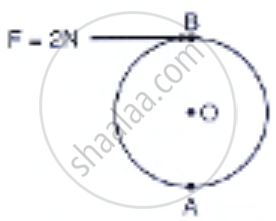Exercise - 1 (A) | Q 4 | Page 11

The diagram in the figure shows two forces F1 = 5 N and F2 = 3N acting at point A and B of a rod pivoted at a point O, such that OA = 2m and OB = 4m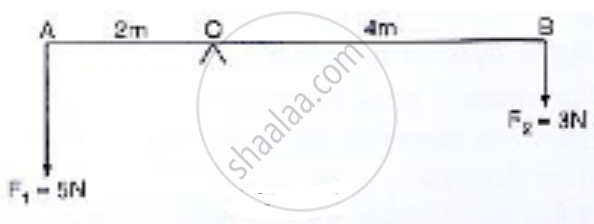Calculate:

1) Moment of force F1 about O

2) Moment of force F2 about O

3) Total moment of the two forces about O.

Exercise - 1 (A) | Q 5 | Page 11

Two forces each of magnitude 10 N act vertically upwards and downwards respectively at the two ends of a uniform road of length 4m which is pivoted at its midpoint as shown in the figure. Determine the magnitude of the resultant moment of forces about the pivot O.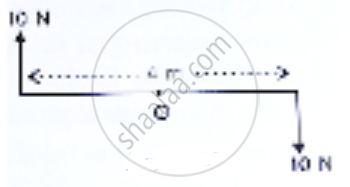Exercise - 1 (A) | Q 6 | Page 11

The figure shows two forces each of magnitude 10 N acting at the point A and B at a separation of 50 cm, in opposite directions. Calculate the resultant moment of two forces about the point A, B and O, situated exactly at the middle of the two forces.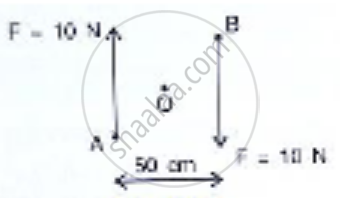Exercise - 1 (A) | Q 7 | Page 11

A steering wheel of diameter 0.5 m is rotated anticlockwise by applying two forces each of magnitude 5 N. Draw a diagram to show the application of forces and calculate the moment of couple applied.

Exercise - 1 (A) | Q 8 | Page 11

A uniform metre rule is pivoted at its mid-point. A weight of 50 gf is suspended at one end of it. where should a weight of 100 gf be suspended to keep the rule horizontal?

Exercise - 1 (A) | Q 9.1 | Page 11

A uniform metre rule balance horizontally on a knife-edge placed at the 58 cm mark when a weight of 20 gf is suspended from one end.

Draw a diagram of the arrangement.

Exercise - 1 (A) | Q 9.2 | Page 11

A uniform metre rule balances horizontally on a knife edge placed at the 58 cm mark when a weight of 20gf is suspended from one end.

What is the weight of the rule?

Exercise - 1 (A) | Q 10 | Page 11

The diagram below Shows a uniform bar supported at the middle point O. A weight of 40 gf is placed at a distance of 40 cm to the left of the point O. How can you balance the bar with a weight of 80 gf?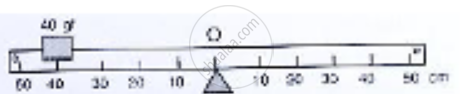Exercise - 1 (A) | Q 11.1 | Page 11

The figure shows a uniform metre rule placed on a function at its mid-point O and having a weight 40 gf at the 10 cm mark and a weight of 20 gf at the 9.0 cm mark.

Is the metre rule in equilibrium? If not how will the rule turn?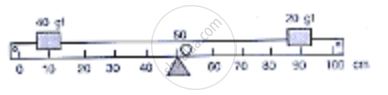Exercise - 1 (A) | Q 11.2 | Page 11

Figure shows a uniform metre rule placed on a fulcrum at its mid-point O and having a weight 40gf at the 10 cm mark and a weight of 20gf at the 90 cm mark.

How can the rule be brought in equilibrium by using an additional weight of 40 gf ?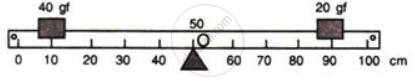Exercise - 1 (A) | Q 12 | Page 12

When a boy weighing 20 kgf sits at one end of a 4 m long see saw, it gets depressed at this end. How can it be brought to the horizontal position by a man weighing 40 kgf

Exercise - 1 (A) | Q 13 | Page 12

A physical balance has its arms of length 60 cm and 40 cm. What weight kept on pan of the longer arm will balance an object of weight 100 gf kept on other pan?

Exercise - 1 (A) | Q 14.1 | Page 12

The diagram in the figure shows a uniform metre rule weighing 100 gf, pivoted as its centre O. two weight 150 gf and  250 gf hang from the point A and B respectively of the metre rule such that OA = 40 cm and OB = 20 cm . Calculate

the total anticlockwise moment about o.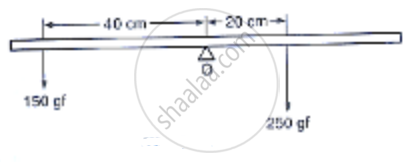Exercise - 1 (A) | Q 14.2 | Page 12

The diagram shows a uniform metre rule weighing 100gf, pivoted at its centre O. Two weights 150gf and 250gf hang from the point A and B respectively of the metre rule such that OA = 40 cm and OB = 20 cm. Calculate :

the total clockwise moment about O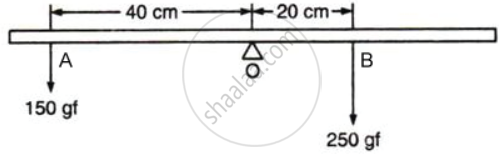Exercise - 1 (A) | Q 14.3 | Page 12

The diagram shows a uniform metre rule weighing 100gf, pivoted at its centre O. Two weights 150gf and 250gf hang from the point A and B respectively of the metre rule such that OA = 40 cm and OB = 20 cm. Calculate :

the difference of anticlockwise and clockwise moment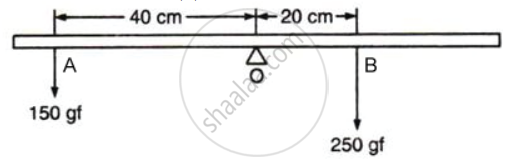Exercise - 1 (A) | Q 14.4 | Page 12

The diagram shows a uniform metre rule weighing 100gf, pivoted at its centre O. Two weights 150gf and 250gf hang from the point A and B respectively of the metre rule such that OA = 40 cm and OB = 20 cm. Calculate :

the distance from O where a 100gf weight should be placed to balance the metre rule.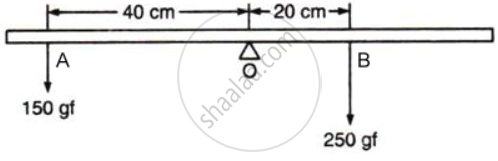Exercise - 1 (A) | Q 15.1 | Page 12

A uniform metre rule of weight 10gf is pivoted at its 0 mark.

What moment of force depresses the rule?

Exercise - 1 (A) | Q 15.2 | Page 12

A uniform metre rule of weight 10gf is pivoted at its 0 mark.

How can it be made horizontal by applying a least force ?

Exercise - 1 (A) | Q 16 | Page 12

A uniform half metre rule can be balanced at the 29.0 cm mark when a mass 20g is hung from its one end.

1) Draw a diagram of the arrangement.

2) Find the mass of the half metre rule.

Exercise - 1 (A) | Q 17.1 | Page 12

A uniform metre rule of mass 100 g is balanced on the fulcrum at mark 10 cm by suspending an unknown mass M at the mark 20 cm.

Find the value of M.

Exercise - 1 (A) | Q 17.2 | Page 12

A uniform metre rule of mass 100g is balanced on a fulcrum at mark 40cm by suspending an unknown mass m at the mark 20cm.

To which side the rule will tilt if the mass m is moved to the mark 10cm ?

Exercise - 1 (A) | Q 17.3 | Page 12

A uniform metre rule of mass 100g is balanced on a fulcrum at mark 40cm by suspending an unknown mass m at the mark 20cm.

What is the resultant moment now ?

Exercise - 1 (A) | Q 17.4 | Page 12

A uniform metre rule of mass 100g is balanced on a fulcrum at mark 40cm by suspending an unknown mass m at the mark 20cm.

How can it be balanced by another mass 50 g ?

Exercise - 1 (A) | Q 18 | Page 12

In following figure , a uniform bar of length l m is supported at its ends and loaded by a weight W kgf at its middle. In equilibrium, find the reactions R1 and R2 at the ends.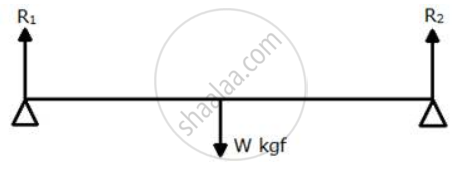["Hint" : "In equilibrium"  "R"_1 + "R"_2 = "W"   "and"   "R"_1 xx 1/2 = "R"_2 xx 1/2]

#### Selina solutions for Concise Physics Class 10 ICSE Chapter 1 Force Exercise Exercise - 1 (B) [Pages 14 - 15]

Exercise - 1 (B) | Q 1 | Page 14

Define the term centre of gravity of a body.

Exercise - 1 (B) | Q 2 | Page 14

Can the centre of gravity be situated outside the material of the body? Give an example

Exercise - 1 (B) | Q 3 | Page 14

On what factor does the position of the centre of gravity of a body depend? Explain your answer with an example.

Exercise - 1 (B) | Q 4.1 | Page 14

What is the position of the centre of gravity of a rectangular lamina?

Exercise - 1 (B) | Q 4.2 | Page 14

What is the position of the centre of gravity of a cylinder?

Exercise - 1 (B) | Q 5.1 | Page 14

At which point is the centre of gravity situated in a triangular lamina.

Exercise - 1 (B) | Q 5.2 | Page 14

At which point is the centre of gravity situated in a circular lamina?

Exercise - 1 (B) | Q 6 | Page 14

Where is the centre of gravity of a uniform ring situated?

Exercise - 1 (B) | Q 7 | Page 14

A square cardboard is suspended by passing a pin through a narrow hole at its one corner. Draw a diagram to show its rest position. In the diagram, mark the point of suspension by the letter S and the centre of gravity by the letter G.

Exercise - 1 (B) | Q 8 | Page 15

Explain how you will determine the position of the centre of gravity experimentally for a triangular lamina (or a triangular piece of cardboard)

Exercise - 1 (B) | Q 9.1 | Page 15

State True or False

The position of the centre of gravity of a body remains unchanged even when the body is deformed.

• True

• False

Exercise - 1 (B) | Q 9.2 | Page 15

State True or False

Centre of gravity of a freely suspended body always lies vertically below the point of suspension.

Exercise - 1 (B) | Q 10 | Page 15

A uniform flat circular rim is balanced on a sharp vertical nai by supporting it at point A, as shown in the figure. Mark the position of the centre of gravity of the rim in the diagram by the letter G.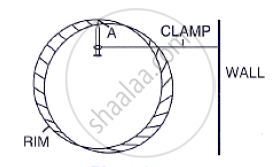Exercise - 1 (B) | Q 11 | Page 15

The figure shows three pieces of cardboard of uniform thickness cut into three different shapes. On each diagram draw two lines to indicate the position of the centre of gravity G.#### Selina solutions for Concise Physics Class 10 ICSE Chapter 1 Force Exercise Exercise - 1 (B) [Page 15]

Exercise - 1 (B) | Q 1 | Page 15

The centre of gravity of a uniform ball is

a) at its geometrical centre

b) at its bottom

c) at its topmost point

d) at any point on its surface

• at its geometrical centre

• at its bottom

• at its topmost point

• at any point on its surface

Exercise - 1 (B) | Q 2 | Page 15

The centre of gravity of a hollow cone of height h is at distance x from its vertex where the value of x is:

• "h"/3

• "h"/4

• "2h"/3

• "3h"/4

#### Selina solutions for Concise Physics Class 10 ICSE Chapter 1 Force Exercise Exercise - 1 (C) [Page 18]

Exercise - 1 (C) | Q 1 | Page 18

Explain the meaning of uniform circular motion. Give one example of such motion.

Exercise - 1 (C) | Q 2 | Page 18

Draw a neat labelled diagram for a particle moving in a circular path with a constant speed. In you diagram show the direction of velocity at any instant.

Exercise - 1 (C) | Q 3 | Page 18

Is it possible to have an accelerated motion with a constant speed? Name such type of motion.

Exercise - 1 (C) | Q 4 | Page 18

Give an example of motion in which speed remains uniform, but the velocity changes.

Exercise - 1 (C) | Q 5 | Page 18

A uniform circular motion is an accelerated motion explain it. State whether the acceleration is uniform or variable? Name the force responsible to cause this acceleration. What is the direction of force at any instant? Draw diagram in support of your answer.

Exercise - 1 (C) | Q 6 | Page 18

Differentiate between Uniform linear motion and  Uniform circular motion.

Exercise - 1 (C) | Q 7 | Page 18

Name the force required for circular motion. State its direction.

Exercise - 1 (C) | Q 8 | Page 18

What is a centripetal force?

Exercise - 1 (C) | Q 9 | Page 18

Explain the motion of a planet around the sun in a circular path.

Exercise - 1 (C) | Q 10.1 | Page 18

With reference to the direction of action, how does a centripetal force differ from centrifugal force?

Exercise - 1 (C) | Q 10.2 | Page 18

Is centrifugal force the force of rection of centripetal force?

Exercise - 1 (C) | Q 10.3 | Page 18

Compare the magnitudes of centripetal and centrifugal force.

Exercise - 1 (C) | Q 11 | Page 18

Is centrifugal force a real force?

Exercise - 1 (C) | Q 12 | Page 18

A small pebble is placed near the periphery of a circular disc which is rotating about an axis passing through it centre.
(a) What will be your observation when you are standing outside the disc? Explain it
(b) What will be your observation when you are standing at the centre of the disc. Explain it

Exercise - 1 (C) | Q 13 | Page 18

A piece of stone tied at the end of a thread is whirled in a horizontal circle. Name the force which provides the centripetal force.

1. Is the velocity of stone uniform or variable?
2. Is the acceleration of stone uniform or variable?
3. What is the direction of acceleration of stone at any instant?
4. What force does provide the centripetal force required for circular motion?
5. Name the force and its direction which acts on the hand.
Exercise - 1 (C) | Q 14 | Page 18

State two differences between the centripetal and centrifugal force.

Exercise - 1 (C) | Q 15.1 | Page 18

State True or False

The earth moves around the sun with a uniform.

• True

• False

Exercise - 1 (C) | Q 15.2 | Page 18

State True or False

The motion of the moon around the earth in a circular path is an accelerated motion.

• True

• False

Exercise - 1 (C) | Q 15.3 | Page 18

State True or False:

A uniform linear motion is unaccelerated , while a uniform circular motion is an accelerated motion.

• True

• False

Exercise - 1 (C) | Q 15.4 | Page 18

State True or False

In a uniform circular motion, the speed continuously changes because of the direction of motion changes.

• True

• False

Exercise - 1 (C) | Q 15.5 | Page 18

State whether the following statement are true or false by writing T/F against them.

A Boy experiences a centrifugal force on his hand when he rotates a piece of stone tied at one end of a string, holding the other end in the hand.

• True

• False

#### Selina solutions for Concise Physics Class 10 ICSE Chapter 1 Force Exercise Exercise - 1 (C) [Pages 0 - 18]

Exercise - 1 (C) | Q 1 | Page 18

Which of the following quantity remains constant in uniform circular motion:

• Velocity

• Speed

• Acceleration

• Both velocity and speed

Exercise - 1 (C) | Q 2

The centrifugal force is:

• a real force

• the force of reaction of centripetal force

• a fictitious force

• directed towards the centre of circular path

## Chapter 1: Force

Exercise -1 (A)Exercise - 1 (A)OthersExercise -1(A)Exercise - 1(A)Exercise - 1 (B)Exercise - 1 (C)## Selina solutions for Concise Physics Class 10 ICSE chapter 1 - Force

Selina solutions for Concise Physics Class 10 ICSE chapter 1 (Force) include all questions with solution and detail explanation. This will clear students doubts about any question and improve application skills while preparing for board exams. The detailed, step-by-step solutions will help you understand the concepts better and clear your confusions, if any. Shaalaa.com has the CISCE Concise Physics Class 10 ICSE solutions in a manner that help students grasp basic concepts better and faster.

Further, we at Shaalaa.com provide such solutions so that students can prepare for written exams. Selina textbook solutions can be a core help for self-study and acts as a perfect self-help guidance for students.

Concepts covered in Concise Physics Class 10 ICSE chapter 1 Force are Turning Forces Concept, Moment of a Force, Forces in Equilibrium, Centre of Gravity, Force - Uniform Circular Motion, Concept of Force, Elementary Introduction of Translational and Rotational Motions, Turning Effect of Force (Moment of Force).

Using Selina Class 10 solutions Force exercise by students are an easy way to prepare for the exams, as they involve solutions arranged chapter-wise also page wise. The questions involved in Selina Solutions are important questions that can be asked in the final exam. Maximum students of CISCE Class 10 prefer Selina Textbook Solutions to score more in exam.

Get the free view of chapter 1 Force Class 10 extra questions for Concise Physics Class 10 ICSE and can use Shaalaa.com to keep it handy for your exam preparation

S